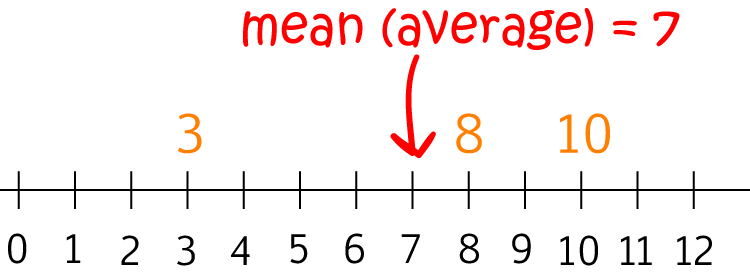# Definition of MeanThe mean of a collection of numbers is their average.

To find the mean, add up the numbers in the group, and then divide the result by the number of numbers in the group.

For example, to find the mean of $3,8$ and $10$:

1. Add the three numbers: $3 + 8 + 10 = 21$.
2. Divide by the number of numbers ($3$): $21 \div 3 = 7$.
So, the mean is $7$.

### Description

The aim of this dictionary is to provide definitions to common mathematical terms. Students learn a new math skill every week at school, sometimes just before they start a new skill, if they want to look at what a specific term means, this is where this dictionary will become handy and a go-to guide for a student.

### Audience

Year 1 to Year 12 students

### Learning Objectives

Learn common math terms starting with letter M

Author: Subject Coach
You must be logged in as Student to ask a Question.